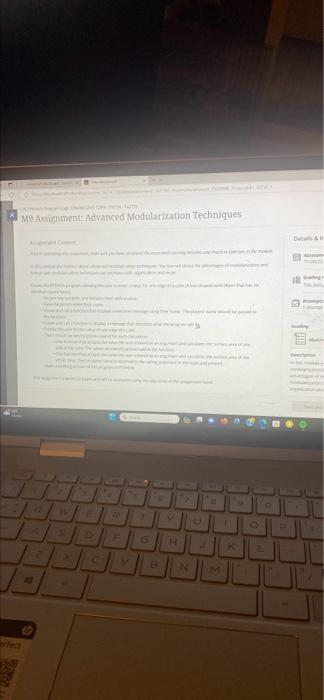In this module you learned about advanced modularization techniques. You learned about the advantages of modularization and how proper modularization techniques can increase code organization and reuse.

Create the PYTHON program allowing the user to enter a value for one edge of a cube (A box-shaped solid object that has six identical square faces).
• Declare any variables and initialize them with a value.
. Have the person enter their name.
• Create and call a function that displays a welcome message using their name. The players' name should be passed to the function.
• Create and call a function to display a message that describes what the program will do.
. Prompt the user for the value of one edge of a cube.
. There should be two functions created for each calculation:
• One function that accepts the value the user entered as an argument and calculates the surface area of one side of the cube. The value calculated is printed within the function.
• One function that accepts the value the user entered as an argument and calculates the surface area of the whole cube. The calculated value is returned to the calling statement in the main and printed.
• Make a working version of this program in PYTHON.We have an Answer from Expert